## Simulate Fuzzy Inference Systems in Simulink

You can simulate a fuzzy inference system (FIS) in Simulink® using either the Fuzzy Logic Controller or Fuzzy Logic Controller with Ruleviewer blocks. Alternatively, you can evaluate fuzzy systems at the command line using `evalfis`.

Using the Fuzzy Logic Controller, you can simulate traditional type-1 fuzzy inference systems (`mamfis` and `sugfis`) and type-2 fuzzy inference systems (`mamfistype2` and `sugfistype2`). The Fuzzy Logic Controller with Ruleviewer block supports only type-1 systems.

For more information on creating fuzzy inference systems, see Build Fuzzy Systems Using Fuzzy Logic Designer and Build Fuzzy Systems at the Command Line.

### Simulate Fuzzy Inference System

Once you have implemented a fuzzy inference system using Fuzzy Logic Designer, using Neuro-Fuzzy Designer, or at the command line, you can simulate the system in Simulink.

For this example, you control the level of water in a tank using a fuzzy inference system implemented using a Fuzzy Logic Controller block. Open the `sltank` model.

```open_system('sltank') ```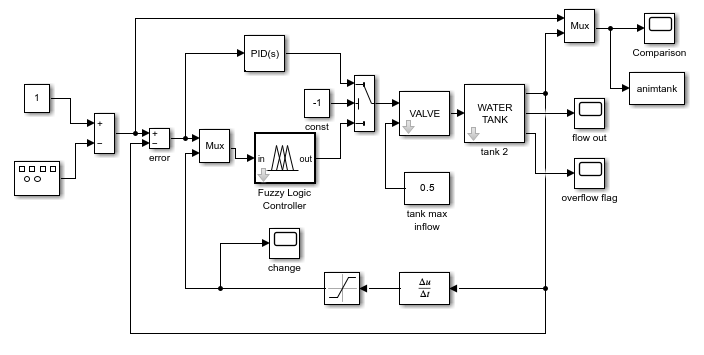For this system, you control the water that flows into the tank using a valve. The outflow rate depends on the diameter of the output pipe, which is constant, and the pressure in the tank, which varies with water level. Therefore, the system has nonlinear characteristics.

The two inputs to the fuzzy system are the water level error, `level`, and the rate of change of the water level, `rate`. The output of the fuzzy system is the rate at which the control valve is opening or closing, `valve`.

To implement a fuzzy inference system, specify the FIS name parameter of the Fuzzy Logic Controller block as the name of a FIS object in the MATLAB® workspace. In this example, the block uses the `mamfis` object `tank`.

For more information on this system, see Water Level Control in a Tank.

As a first attempt to control the water level, set the following rules in the FIS. These rules adjust the valve based on only the water level error.

• If the water level is okay, then do not adjust the valve.

• If the water level is low, then open the valve quickly.

• If the water level is high, then close the valve quickly.

Specify the rules by creating a vector of `fisrule` objects and assigning it to the `Rules` property of the `tank` FIS object.

```rule1 = "If level is okay then valve is no_change"; rule2 = "If level is low then valve is open_fast"; rule3 = "If level is high then valve is close_fast"; rules = [rule1 rule2 rule3]; tank.Rules = fisrule(rules); ```

Simulate the model, and view the water level.

```open_system('sltank/Comparison') sim('sltank',100) ```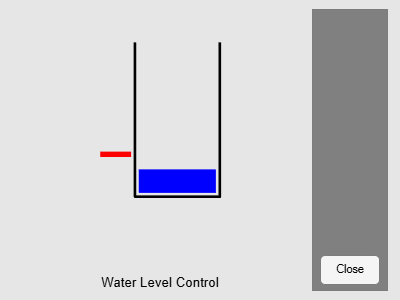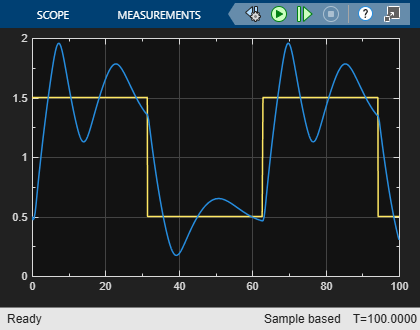These rules are insufficient for controlling the system, since the water level oscillates around the setpoint.

To reduce the oscillations, add two more rules to the system. These rules adjust the valve based on the rate of change of the water level when the water level is near the setpoint.

• If the water level is okay and increasing, then close the valve slowly.

• If the water level is okay and decreasing, then open the valve slowly.

To add these rules, use the `addRule` function.

```rule4 = "If level is okay and rate is positive then valve is close_slow"; rule5 = "If level is okay and rate is negative then valve is open_slow"; newRules = [rule4 rule5]; tank = addRule(tank,newRules); ```

Simulate the model.

```sim('sltank',100) ```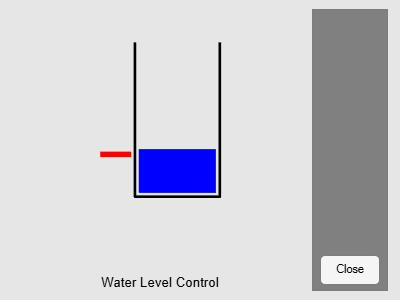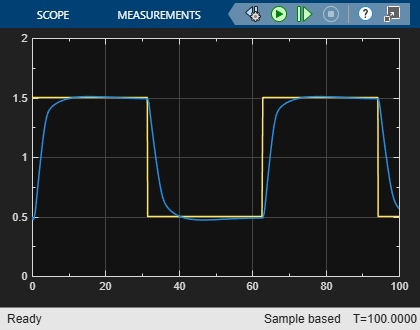The water level now tracks the setpoint without oscillating.

You can also simulate fuzzy systems using the Fuzzy Logic Controller with Ruleviewer block. The `sltankrule` model is the same as the `sltank` model, except that it uses the Fuzzy Logic Controller with Ruleviewer block.

```open_system('sltankrule') ```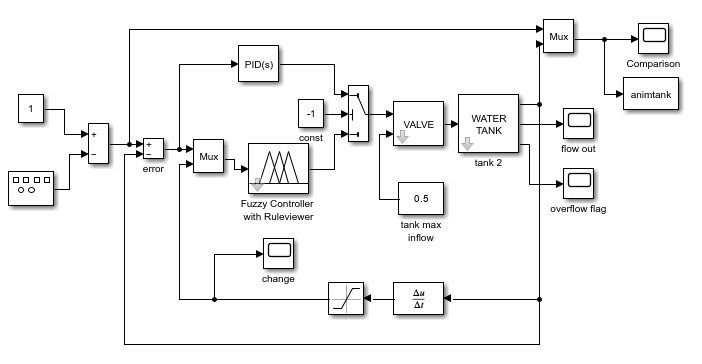During simulation, this block displays the Rule Viewer from the Fuzzy Logic Designer app.

```sim('sltankrule',100) ```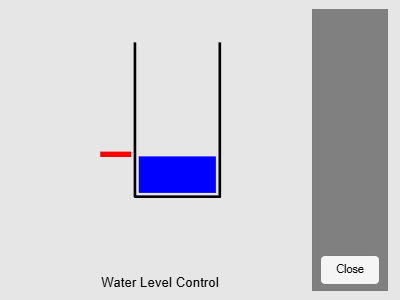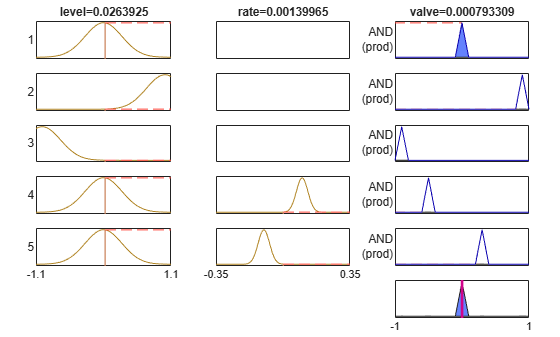If you pause the simulation, you can examine the FIS behavior by manually adjusting the input variable values in the Rule Viewer, and observing the inference process and output.

You can also access the Fuzzy Logic Designer editors from the Rule Viewer. From the Rule Viewer, you can then adjust the parameters of your fuzzy system using these editors, and export the updated system to the MATLAB workspace. To simulate the updated FIS, restart the simulation. For more information on using these editors, see Build Fuzzy Systems Using Fuzzy Logic Designer.

### Access Intermediate Fuzzy Inference Results

You can access intermediate fuzzy inference results using the Fuzzy Logic Controller block. You can use this data to visualize the fuzzy inference process or troubleshoot the performance of your FIS. To access this data, enable the corresponding parameters in the block, and connect signals to the corresponding output ports.

Block Parameter DescriptionOutput Port
Fuzzified InputsFuzzified input values, obtained by evaluating the input membership functions of each rule at the current input values.`fi`
Rule firing strengthsRule firing strengths, obtained by evaluating the antecedent of each rule.`rfs`
Rule outputsRule outputs, obtained by evaluating the consequent of each rule.`ro`
Aggregated outputsAggregate output for each output variable, obtained by combining the corresponding outputs from all the rules.`ao`

### Simulation Modes

The Fuzzy Logic Controller block has the following two simulation modes:

• `Interpreted execution` — Simulate fuzzy systems using precompiled MEX files. Using this option reduces the initial compilation time of the model.

• `Code generation` — Simulate fuzzy system without precompiled MEX files. Use this option when simulating fuzzy systems for code generation applications. Doing so simulates your system using the same code path used for generated code.

To select a simulation mode, set the Simulate using parameter of the block. By default, the block uses `Interpreted execution` mode for simulation.

### Map Command-Line Functionality to Fuzzy Logic Controller Block

The parameters and ports of the Fuzzy Logic Controller block map to the input and output arguments of `evalfis` or the properties of `evalfisOptions`. The following table shows the block parameters and ports that map to `evalfis` arguments.

`evalfis` ArgumentDescriptionBlock Parameter or Port
`fis`Fuzzy inference systemFIS name
`input`, when a single rowInput variable values`in`
`output`, when a single rowOutput variable values`out`
`fuzzifiedIn`Fuzzified inputs`fi`
`ruleOut`Rule outputs`ro`
`aggregateOut`Aggregated outputs`ao`
`ruleFiring`Rule firing strengths`rfs`

The following table shows the block parameters that map to `evalfisOptions` properties.

`evalfisOptions` PropertyDescriptionBlock Parameter or Port
`NumSamplePoints`Number of points in output fuzzy setsNumber of samples for output discretization
`OutOfRangeInputValueMessage`Diagnostic message behavior when an input is out of rangeOut of range input value
`NoRuleFiredMessage`Diagnostic message behavior when no rules fireNo rule fired
`EmptyOutputFuzzySetMessage`Diagnostic message behavior when an output fuzzy set is emptyEmpty output fuzzy set

The remaining parameters of the Fuzzy Logic Controller block do not map to arguments of `evalfis`. Also, unlike the Fuzzy Logic Controller block, `evalfis` does not support fixed-point data for simulation or code generation.